#Microsoft Access Financial Cash Flow Calculations with Total Access Statistics

Microsoft Access does not include financial functions to analyze cash flow data in your tables. Performing such calculations has been quite challenging for MS Access users and developers. Fortunately, Total Access Statistics performs a wide range of cash flow analysis:

• For periodic payments and receipts, use discounted cash flows to generate Future Value (FV), Net Present Value (NPV), Internal Rate of Return (IRR), and Modified Internal Rate of Return (MIRR)
• For date dependent payments and receipts, use discounted cash flows to generate Future Value (FV), Net Present Value (XNPV), Internal Rate of Return (XIRR), and Modified Internal Rate of Return (XMIRR)
• Analyze the data in your Access tables and queries (including linked data)
• Specify Group By fields to perform multiple analyses in one pass (one result for each unique combination of group fields)
• Generate results in Access tables that you can use in your queries, forms, and reports

Best of all, by using a database approach with support for groupings, calculations for each group of records is automatically generated making it far more powerful than performing the calculations in Microsoft Excel. Since the results are in Access tables, you can add them to your forms and reports.

Financial Calculations were added to Total Access Statistics for Microsoft Access 2010, and X.8 versions for Access 2007 and earlier.

## Financial Analysis Overview

If you have a table with records containing your cash flow information, Total Access Statistics can perform the financial analysis you seek. Easily calculate discounted values and returns for a series of cash flow investments and receipts. Negative values are considered investments (payments) and positive values are receipts (returns).

Two Financial scenario types are available depending on whether your data represents Periodic or Irregular cash flows:

• Periodic Cash Flows
Each record is a cash flow payment or receipt that is identically separated in time from each other (for instance, annual or monthly transactions), but the amount may vary for each period.
• Irregular Cash Flows (Date Dependent)
Each record has a date field for its cash flow payment or receipt which may not be evenly spaced over time. The amounts may also vary with each record.

## Periodic Cash Flows

The simplest and most common cash flow analysis is based on periodic transactions. Payments or receipts can vary in size but occur on a regular schedule. Most common intervals are annual, monthly, and quarterly. Depending on the frequency of your cash flows, you’d enter the appropriate interest rate for each period.

### Periodic Cash Flow Field Selection

The following field selection screen appears for Periodic Cash Flows: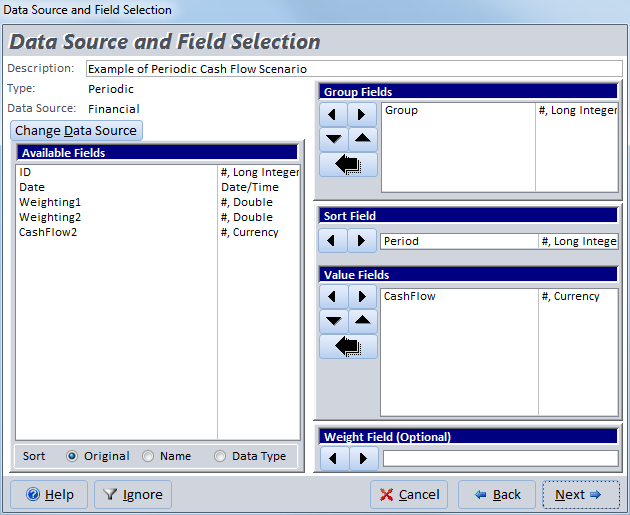The Value Fields contain the cash flow data. Each field you selected is analyzed separately. The values in the field should be negative for payments (investments or outflows), and positive for receipts (returns or inflows).

Group Fields let you generate a separate set of analysis for each combination of unique values among the group fields.

The Sort Field is used to ensure your cash flows are sorted properly. This may be the AutoNumber field of your table or another field that can be used to ensure Total Access Statistics processes each cash flow in the right order. It is critical that your cash flows be in the right order.

If no group field is specified, the Sort Field is not required and Total Access Statistics analyzes your records in their natural order, which may or may not be okay. You need to verify this.

You can optionally specify a Weight Field which can be used in situations where you have a field for the receipt amount and another weighting field that specifies the quantity of those receipts.

### Periodic Cash Flow Options

After selecting the fields, the Periodic options are presented: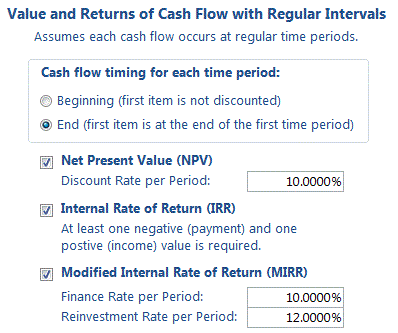#### Cash flow timing for each time period

For Cash Flow analysis, it’s important to determine whether the first item represents an event at the beginning of the time period or at the end of the time period. For instance, if your initial investment (a negative number) occurs on Day 1, choose the Beginning option. If the first investment occurs at the end of the first year, choose End.

The implication of this selection can be most easily understood with the first investment. If it’s at the beginning of the period, the first cash flow is not discounted since there hasn’t been any time to apply the interest rate. If it’s at the end, it is adjusted by the discount rate.

#### Net Present Value (NPV)

Net Present Value is the current value of a future series of payments and receipts and a way to measure the time value of money. Basically, money today is worth more than money tomorrow. And future money is discounted by the interest rate you specify.

Assuming cash flows occur at the end of each period, an NPV with a 10% discount rate would divide the cash flow of period 1 by (1 + 10%) then add the cash flow in period 2 divided by (1 + 10%) ^2, etc. The NPV calculation ends with the last cash flow. The formula for NPV is: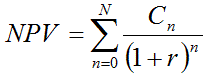where N is the number of periods, n is a specific period, C is the cash flow for a particular period and r is the discount rate for each period.

#### Set the Discount Rate to Your Time Interval

The discount rate you enter must correspond to the period of time between each cash flow. For periodic cash flow analysis, Total Access Statistics does not have date information on when the events occur.

If it’s monthly, you should use 1/12th of your annual rate, for quarterly one-fourth, etc. For most accurate results, take the nth root of your annual discount rate to capture the compounding effect.

### Internal Rate of Return (IRR)

The Internal Rate of Return is used to measure an investment's attractiveness. It is the interest rate that makes the NPV equal to zero for the series of cash flows. At least one negative payment and one positive receipt are required to calculate IRR. If this doesn’t exist, the result is null.

IRR is sometimes called the discounted cash flow rate of return, rate of return, and effective interest rate. The “internal” term signifies the rate is independent of outside interest rates.

Depending on the number of cash flows and their values, IRR can require many iterations to generate an accurate result. Microsoft Excel stops after 20 tries. Total Access Statistics generates its standard level of double precision accuracy and will do so for up to 1000 iterations.

### Modified Internal Rate of Return (MIRR)

Modified Internal Rate of Return is used to measure an investment's attractiveness. MIRR is a modification of the IRR calculation and resolves some problems with the IRR.

IRR assumes that positive cash flows are reinvested at the same rate of return as that of the investment. This is unlikely as funds are reinvested at a rate closer to the organization’s cost of capital or return on cash. The IRR therefore often gives an overly optimistic rate of the cash flows. For comparing projects more accurately, the cost of capital should be used for reinvesting the interim cash flows.

Additionally, for projects with alternating positive and negative cash flows, more than one IRR may be found, which may lead to confusion.

To use MIRR, provide the two interest rates:

• Finance Rate: the cost of capital
• Reinvestment Rate: the interest received for cash investments

Similar to the discount rate provided for NPV, these rates should be the rate for each period and not the annual rates if your periods are not yearly. The formula for MIRR is: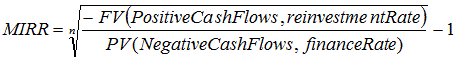where n is the number of equal periods at the end of which the cash flows occur (not the number of cash flows), PV is present value (at the beginning of the first period), FV is future value (at the end of the last period).

MIRR sums the discounted negative cash flows to the starting time, and sums the positive cash flows to the final period adjusting for the reinvestment rate. By dividing and taking the nth root, it determines the rate of return for the positive and negative cash flows.

Note that in Excel or VBA, the MIRR function always assumes the cash flows are at the beginning of the period. If you want to use the End of period option in Total Access Statistics and compare it to Excel, add an extra cash flow of zero to the beginning of the Excel data set.

### Periodic Cash Flow Output Table

Periodic Cash Flow analysis is performed for each value field: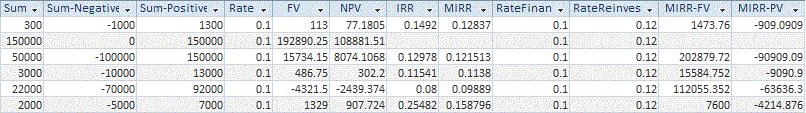Example of Periodic Cash Flow Analysis Results in a Microsoft Access table

### Periodic Cash Flow Output Fields

These fields are calculated for all periodic cash flow analysis:

Field Name Description
Group Fields Group fields selected (if any)
DataField Field name identifying the data in the record
Count (N) Number of records in the group
Missing Records with missing values
FirstCashFlow Value of the first cash flow
LastCashFlow Value of the last cash flow
Sum Sum of the values (profit)
Sum-Negative Sum of the negative cash flows (values less than zero)
Sum-Positive Sum of the positive cash flows (values greater than zero)
These fields are created if the NPV option is selected
Rate The discount rate you specified for each period
FV The Future Value of the cash flows at the end of the last period
NPV Net Present Value of the discounted cash flows
This field is created if the IRR option is selected
IRR Internal Rate of Return
These fields are created if the MIRR option is selected
MIRR Modified Internal Rate of Return
RateFinance The periodic finance rate you specified
RateReinvest The periodic reinvestment rate you specified
MIRR-PV The present value of the negative cash flows discounted by the finance rate
MIRR-FV The future value of the positive cash flow discounted by the reinvestment rate

As with all other values generated by Total Access Statistics, the rates (percentages) are shown as a decimal value between 0 and 1 (e.g. 5% is 0.05).

## Irregular, Date Dependent Cash Flows

Irregular Cash Flow analysis is similar to Periodic Cash Flow analysis, except the timing of individual cash flows is taken into account.

Periodic analysis assumes the same amount of time between each cash flow. For situations where you have dates associated with each cash flow, the irregular cash flow analysis will provide a more accurate result.

### Irregular Cash Flow Field Selection

The following field selection screen appears when you choose Irregular Cash Flow analysis: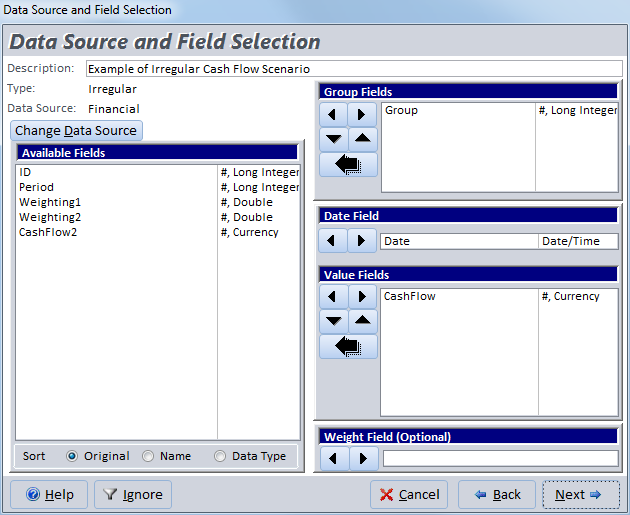### Irregular Cash Flows Field Selection

This is similar to the selection for Periodic Cash Flows, except a Date Field is required to identify the timing of each cash flow.

### Irregular Cash Flow Options

After selecting the fields, the Irregular options are presented: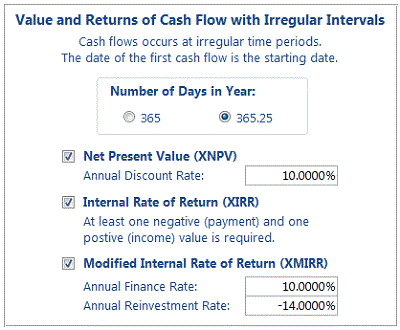### Number of Days in Year

To adjust the interest rate to apply to cash flows between two dates, the annual interest rate needs to be divided by the number of days in a year.

365 days is the most common selection but if your cash flows span over multiple years, using 365.25 to adjust for leap year is more accurate. The difference of course is rather small.

### Net Present Value (XNPV)

Net Present Value is the current value of a future series of payments and receipts and a way to measure the time value of money. This is identical to what’s calculated in the Periodic cash flow analysis. The difference in the irregular cash flow analysis is that each cash flow is adjusted by the overall annual discount rate based on the date it occurs. NPV where dates are taken into account is called XNPV.

Unlike the periodic cash flow analysis where you specify the discount rate for each period, the discount rate for irregular cash flow analysis is always the annual rate.

### Internal Rate of Return (XIRR)

The Internal Rate of Return is the interest rate that makes the NPV equal to zero for the series of cash flows. At least one negative payment and one positive receipt are required to calculate IRR. If this doesn’t exist, the result is null.

This is identical to what’s calculated in the periodic cash flow analysis. The difference in the irregular cash flow analysis is that each cash flow is specific to the date it occurs. IRR where dates are taken into account is called XIRR.

### Modified Internal Rate of Return (XMIRR)

MIRR is a modification of the IRR calculation and is a more accurate reflection of the true rate of return for a series of cash flows. MIRR where dates are taken into account is called XMIRR. To calculate XMIRR, provide the two interest rates:

• Finance Rate: the cost of capital
• Reinvestment Rate: the interest received for cash investments

Unlike periodic cash flows, the rates for irregular cash flows are always the annual rate.

### Irregular Non-Periodic Cash Flow Output Table

Irregular Non-Periodic Cash Flow analysis is performed for each value field: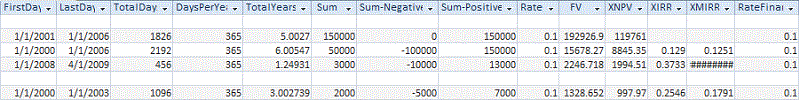Example of Non-Periodic Cash Flow Analysis Results in a Microsoft Access table

## Interactive Wizard and VBA Programmatic Interfaces

Total Access Statistics lets you interactively generate your calculations through its wizard interface to easily select the data source, fields, and analysis without programming. After you specify your selections, they are automatically saved as a scenario in your database so you can run them again in the future when your data changes.

A VBA statistics function is also available so you can generate these results from code. You can easily run any saved scenario by calling a single procedure in the Total Access Statistics VBA library. The library includes a royalty-free runtime license so you can include it with your Access database and distribute it to non-Total Access Statistics owners.

Call the function from a button's OnClick event or other process, and the results are generated for you. You can even hide the built-in user interface so your users don't even know Total Access Statistics is running. They'll be amazed with your statistical analysis capabilities!

## Additional Details of Calculations Available in Total Access Statistics

Here are some additional resources and details about some of the data analysis you can perform on your Microsoft Access data with Total Access Statistics:

## Total Access Statistics

### Microsoft Access 2016 and 2013 Versions are shipping!

Version 14.1 for
Microsoft Access 2010

Version X.9 for
Microsoft Access 2007 and 2003

Version X.8 for
Microsoft Access 2002, 2000, and 97

### Reviews

"Total Access Statistics performs a wide range of data analysis right inside Access. The product includes a Statistics Wizard to perform parametric, group, non-parametric, record analysis and more. The results are placed in Access tables that you can integrate into your forms and reports."

Alison Balter, Author

More Awards Reviews and References in Scientific Papers

### Statistics Info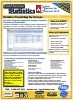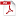PDF Factsheet